# Accessing the Raspberry Pi camera image sensor

WARNING You can easily ruin your Raspberry Pi camera module by following the steps in this post. Proceed with caution.

Over the past half year I have been making slow but steady progress on my lensless imager project. The purpose, aside from having a bit of fun, is to create an imaging system for basic cell biology that doesn't use an expensive microscope objective.

A lensless imager works just as its name implies: an image sensor records the scattered light from a microscopic, transparent object and computationally reconstructs an image of that object, all without a lens. The best resolutions are achieved when the object is relatively close to the image sensor. Ideally, the separation between the object and the sensor's pixels would be at most about one millimeter. This limit is partly determined by the light source's spatial coherence, but also by the fact that high resolution is achieved by recording the scattered light at very large angles, which is possible only when the sample is close to the sensor.

Today I had a bit of free time so I decided to see whether I could remove the housing that surrounds the Raspberry Pi camera's sensor. The Raspberry Pi Camera Module version 2 sensor is a Sony IMX219 color chip. Directly above the sensor is a filter, a lens, and the housing for both of these that prevent me from placing anything closer than about half a centimeter from the sensor plane. If I would want to use this camera for the lensless imager, then the housing would have to go.

Now, even without the housing the Raspberry Pi camera is not necessarily the best option for the project because the IMX219 is a color sensor. This means that there is a Bayer filter over its pixels, which would cut the resolution of the imager since I would be using a very narrow band light source. Effectively, only a quarter of the pixels would be receiving any light. Regardless, I had a spare second camera and it interfaces well with the Raspberry Pi, so I figured it would make for a good prototype.

As you will see, I did slightly damage my sensor, though it seems to still work. You can easily ruin your camera module or cut your finger by following these steps, so proceed at your own risk.

## Step 0: The Raspberry Pi Camera Module V2

In the picture below you see my Raspberry Pi Camera Module. From above, you can see the small circular aperture with the lens immediately behind it. The sensor is inside the small gray rectangular housing that is attached to the control board by a small ribbon cable (to its right) and a bit of two-sided sticky foam tape (underneath the sensor; not visible).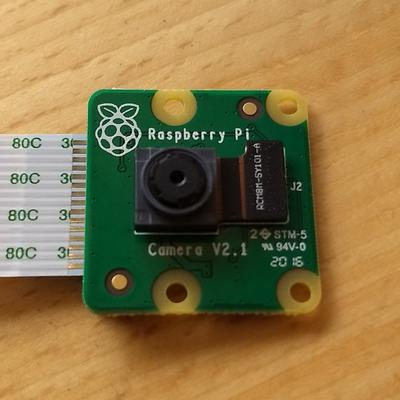## Step 1: Remove the main ribbon cable

To make working on the board a bit easier, I removed the white ribbon cable that attaches the module to the Pi. I did this by pulling on the black tabs on the two ends of the connecter until the cable is easily removed. I labeled the sides of the ribbon cable just in case.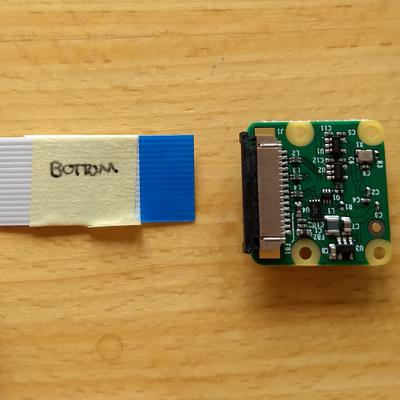## Step 2: Detach the sensor's ribbon cable

Next, I used my finger and thumb nail to remove the small ribbon cable that attaches the sensor to the control board. I essentially applied a small torque to the bottom edge of the connector until it just "popped" up, as seen in the second image below.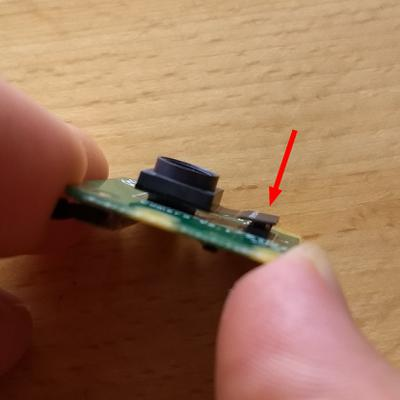## Step 3: Remove the sensor from the control board

In the third step, I used my thumbnail to gently pry the sensor from the control board. The sensor is attached with some two-sided sticky tape and may need a few minutes of work to come free.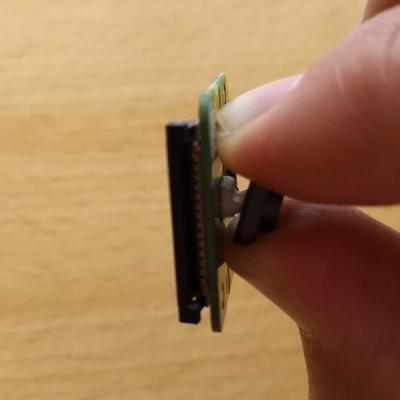## Step 4: Remove the rectangular housing

In this step you risk cutting your finger, so please be careful.

The housing around the sensor is glued. To remove it, you will need to gently work a knife (or, better yet, a thin screw driver) between the housing and the sensor board, taking care not to let the blade go too far into the housing and possibly ruining one of the resistors or wire bonds.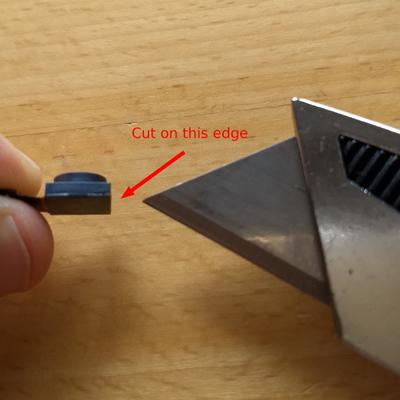Once you get a knife between the two, try popping the housing off of the sensor.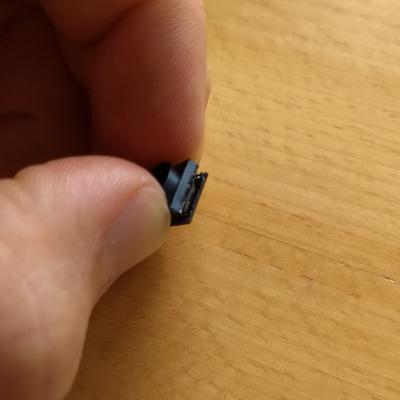When I did this I cut on three sides of the housing, but in retrospect I should have only cut on the side opposite the ribbon cable and pried the other sides loose. This is because I damaged a small resistor when the knife blade went too far into the housing. You can see this below and, at the same time, get an idea of the layout of the sensor board so you know where you can and can't cut.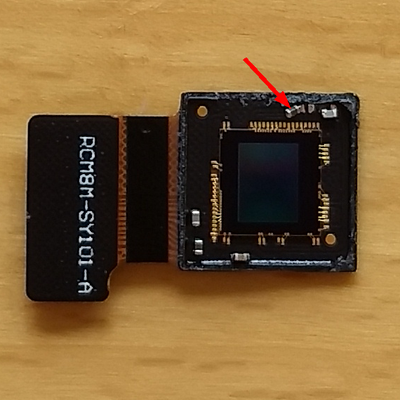If you have the normal version of the camera, then you can also find the IR blocking filter inside the housing.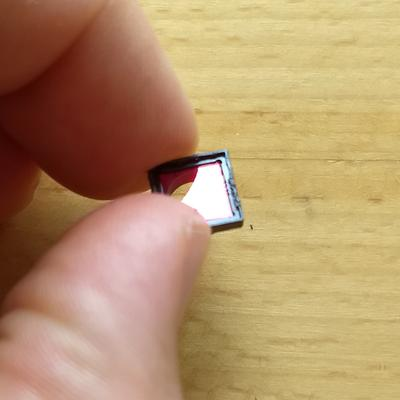Fortunately for me the camera still works, despite the damaged resistor. I can now place samples directly on the sensor if I wanted to, though the wire bonds from the sensor to its control board appear quite fragile. For this reason, it may make more sense to build a slide holder that holds a sample just above the surface without touching it. For now, I can use this exposed sensor to prototype different methods for mounting the sample.

# Modeling noise for image simulations

In my previous post, I described a method for simulating inline holography images. The ultimate purpose of doing this was to generate holography data that could be used to test the performance of different reconstruction algorithms. Since I would know the input object, I could apply the reconstruction algorithm to the holograms and see how closely the reconstructed object matched the known input.

My modeling however ignored an important aspect about the holographic process, namely the acquisition of digital images and the assoicated noise that would accompany the raw data. This is important because holographic reconstruction algorithms need to be robust against the uncertainty that will inevitably be introduced by recording the hologram with a digital camera.

In :
%pylab inline
import scipy
from numpy.fft import fft2, fftshift, ifftshift
from mpl_toolkits.axes_grid1 import make_axes_locatable
from matplotlib import animation
plt.style.use('dark_background')
plt.rcParams['figure.facecolor'] = '#272b30'
plt.rcParams['image.cmap'] = 'viridis'

print('Python version:\n{}\n'.format(sys.version))
print('Numpy version:\t\t{}'.format(np.__version__))
print('matplotlib version:\t{}'.format(matplotlib.__version__))
print('Scipy version:\t\t{}'.format(scipy.__version__))

Populating the interactive namespace from numpy and matplotlib
Python version:
3.7.0 (default, Aug 17 2018, 19:20:07)
[GCC 5.4.0 20160609]

Numpy version:		1.16.1
matplotlib version:	3.0.2
Scipy version:		1.2.1


## A Mathematical Model for Camera Noise¶

One of the best descriptions of camera noise that I have ever found may be the EMVA 1288 Standard. This document details a standard set of models and procedures to characterize CMOS and CCD cameras and light sensors. From its website:

EMVA launched the initiative to define a unified method to measure, compute and present specification parameters for cameras and image sensors used for machine vision applications... [The EMVA Standard] creates transparency by defining reliable and exact measurement procedures as well as data presentation guidelines and makes the comparison of cameras and image sensors much easier.

In particular, chapters 2 and 3 of the Standard provide a very clear and concise description the mathematical model for CCD/CMOS camera noise. Below, I have adapted the image of the mathematical model presented at the beginning of chapter 2.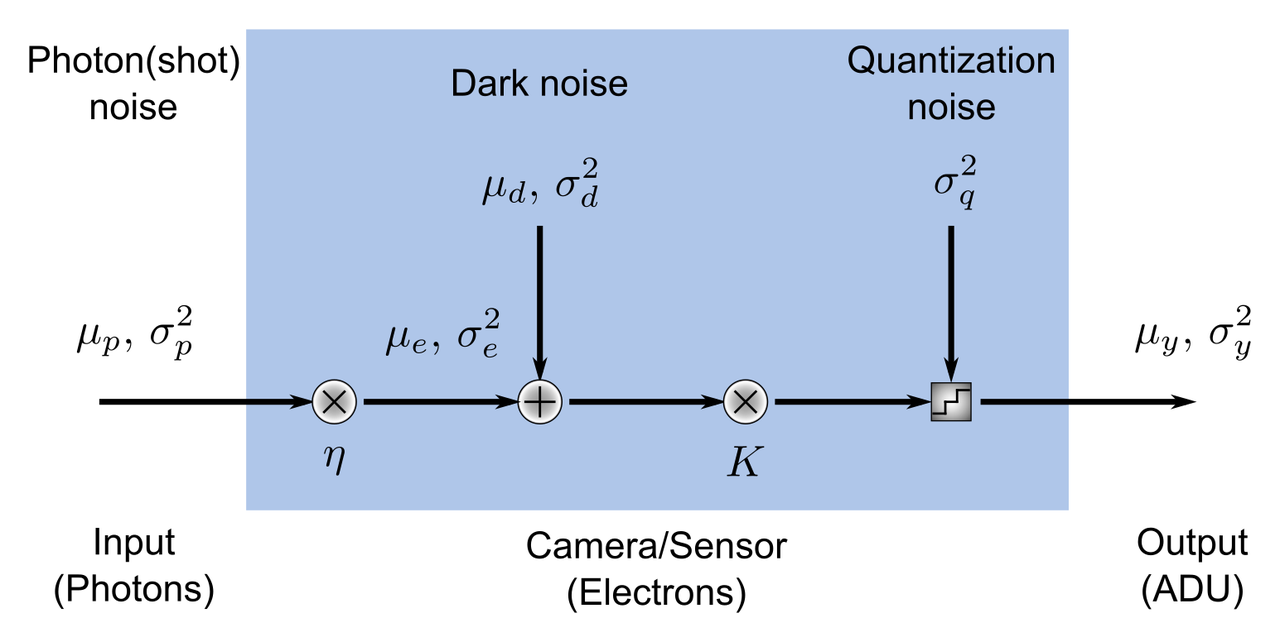In this model, the camera/sensor is a single pixel that takes an input (a number of photons) and transforms this number to an output (a digital value in analog-to-digital units, or ADU). In performing this transformation, various sources of noise are added to the signal so that, given a camera's output in ADU, we can only make probabilisitic inferences about the actual number of photons impinging upon it. There are also a few assumptions in this model that, when taken together, constitute what's called an ideal image sensor. They are:

1. the numer of photons collected by the pixel depends on the product of irradiance $E \, \left[ W / m^2 \right]$ and the exposure time $t_{exp} \, \left[ s \right]$;
2. the sensor is linear, i.e. the digital signal $y$ increases linearly with the number of photons received;
3. all noise sources are constant in time and space;
4. only the total quantum efficiency of the camera is dependent on the wavelength of the incident irradiation;
5. and only the dark current depends on the temperature.

From these five assumptions, the laws of quantum mechanics, and the laws of probability, expressions for the mean output, $\mu_y$, the variance of the output $\sigma_y^2$, and the signal-to-noise ratio ($SNR$) may be derived.

\begin{equation*} \mu_y = K \mu_d + K \eta \mu_p \\ \sigma_y^2 = K^2 \sigma_d^2 + K \left( \mu_y - K \mu_d \right) \\ SNR = \frac{\eta \mu_p}{\sqrt{\sigma_d^2 + \sigma_q^2/K^2 + \eta \mu_p}} \end{equation*}

In these expressions, $\mu$ and $\sigma^2$ represent the mean and variance of the number of photons hitting the camera ($p$), the dark noise ($d$), and the output signal in ADU's ($y$). The number of electrons $\mu_e$ generated by $\mu_p$ photons hitting the active area of the sensor is obtained from the expression for the quantum efficiency $\eta$, an engineered property of the camera that in general depends on the wavelength.

\begin{equation*} \eta \left( \lambda \right) = \frac{\mu_e}{\mu_p} \end{equation*}

The sensitivity of the camera $K \, \left[ ADU / e^- \right]$ represents the amplification of the voltage in the pixel from the photoelectrons and is also a property of the camera. Finally, $\sigma_q^2$ represents the noise introduced by digitzing the continous voltage signal into a digital one.

The EMVA 1288 Standard explains how to derive these expressions from the assumptions and known physical laws, so I will not go into their derivations in this post. Instead, I will focus on explaining how we can use this model to simulate the effects of camera noise in our holograms.

## From Model to Simulation¶

The goal of the simulation will be to take a simulated hologram as input and convert it into an image that might be captured by a digital camera. This means that the final image should contain noise that is realistic and follows the rules of the model.

The model will depend on five free parameters that vary from camera-to-camera. They are:

1. the total quantum efficiency $\eta$;
2. the read noise variance $\sigma_{r}^2$ (or its standard deviation);
3. the dark current $\mu_I$;
4. the sensitivity $K$;
5. and the bit-depth of the camera $k$.

The dark noise $\sigma_d^2$ is actually comprised of two separate noise sources, i.e. the read noise $\sigma_r^2$ and the dark current $\mu_I$. Because the noise sources are independent, we can write

\begin{equation*} \sigma_d^2 = \sigma_r^2 + \sigma_I^2 \\ \sigma_d^2 = \sigma_r^2 + \mu_I t_{exp} \end{equation*}

where the last step relies on the fact that the generation of thermal electrons is a Poisson process whose mean is equal to its variance. The units of $\mu_I$ are $e^- / s$ and values for $\mu_I$ are often provided on camera spec. sheets. Likewise, the read noise $\sigma_r^2$ is also provided on spec. sheets, although in my experience the units are likely to be in root-mean-square electrons or median electrons and not electrons-squared.

Other than the inputs, we do not need to provide values for the other numbers in the model because they are related to the free parameters by various physical and mathematical laws.

Finally, let's ignore spatial noise sources in these simulations because it's difficult to characterize them with a single number. Spatial noise is a variation in the free parameters of the camera from pixel to pixel. An example of a common type of spatial noise is photon response non-uniformity and is manifest in a sensitivity $K$ that varies between pixels. Ignoring spatial noise in the simulations simply means that values for the read noise, dark current, quantum efficiency, and sensitivty are the same for all pixels.

### Step 0: Convert the electric field to photons¶

Before doing anything, we need to take the electric field from our Fourier optics simulations and convert it to units of photons. If it isn't clear how you would first get an electric field, you can refer to my previous post on calculating the hologram of a thin, circular phase-only object. Typically, the calcuation of the electric field will follow from the laws of diffraction and serve as an input for the camera model.

To get the number of photons, we first compute the irradiance of the field using the magnitude of the time-averaged Poynting vector of a linearly-polarzed electromagnetic wave in free space, which is

\begin{equation*} E \left(x, y \right) = \frac{1}{2Z_o} \left| U\left(x, y \right) \right|^2 \end{equation*}

Here, $E \left( x, y \right)$ is the irradiance at point $\left( x, y \right)$, $U \left( x, y \right)$ is the scalar electric field at the same point, and $Z_0 = 377 \, \Omega$ is the impedance of free space. We would change the value for the impedance if we were imaging in a medium other than vacuum. The units of the field are $V / m$ which, when squared and divided by the units of the impedance (Ohms), gives units of power per area, or $W / m^2$. It's also worth mentioning that we are implicitly assuming the field varies smoothly on the length scale of a pixel. This is why we can use the expression for the Poynting vector of a plane wave.

The mean number of photons is obtained from the irradiance by the expression

\begin{align*} \mu_p &= \frac{\lambda A}{hc}Et_{exp} \\ &= \frac{\lambda A}{2hcZ_0} \left| U \right|^2 t_{exp} \end{align*}

where $A$ is the pixel's area and $\frac{hc}{\lambda}$ is the energy per photon in units of Joules, with $hc = 2 \times 10^{-25} \, J \cdot m$.

This step is a bit cumbersome, and in the rest of what follows I will skip this step and will use a set number of photons directly as the input to the simulations, rather than a scalar field.

### Step 1: Compute the photon shot noise¶

For the sake of simplicity, let's start by assuming that we have a camera with $256 \times 256$ pixels and a mean incident photon flux of 500 photons on each pixel. This would produce an image that looks like this:

In :
num_photons = 500
num_pixels  = 256
mu_p        = num_photons * np.ones((num_pixels, num_pixels))

fig, ax = plt.subplots()
img = ax.imshow(mu_p, vmin=400, vmax=600)
ax.set_xticks([])
ax.set_yticks([])
ax.set_title('No noise')

cb = plt.colorbar(img)
cb.set_label('Photons')

plt.show()Photon shot noise arises from the fact that the incident number of photons is not deterministic but rather a random process whose statistics are Poissonian. In a Poisson process, the variance of the noise $\sigma_p^2$ is equal to the mean number of photons $\mu_p$.

Poisson random numbers are easy to generate with NumPy. First, we set a seed so that we can reproducibly generate the same random numbers each time and initialize a RandomState instance with it. Using this RandomState instance, we call the poisson method with a mean of num_photons and a size (num_pixels, num_pixels). Finally, we plot the image and compare it to the one where no shot noise is present.

In :
seed       = 42
rs         = np.random.RandomState(seed)
shot_noise = rs.poisson(num_photons, (num_pixels, num_pixels))

fig, (ax0, ax1) = plt.subplots(ncols=2)
img0 = ax0.imshow(mu_p, vmin=400, vmax=600)
ax0.set_xticks([])
ax0.set_yticks([])
ax0.set_title('No shot noise')

divider = make_axes_locatable(ax0)
cb0 = plt.colorbar(img0, cax=cax)
cb0.set_ticks([])

img1 = ax1.imshow(shot_noise, vmin=400, vmax=600)
ax1.set_xticks([])
ax1.set_yticks([])
ax1.set_title('Shot noise')

divider = make_axes_locatable(ax1)
cb1 = plt.colorbar(img1, cax=cax)
cb1.set_label('Photons')

plt.show()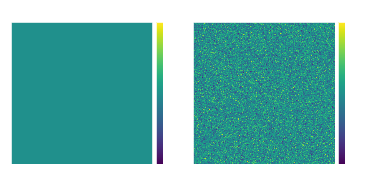In :
# It's also interesting to look at the distribution of photons hitting each pixel.
plt.hist(shot_noise.ravel(), bins=np.arange(350, 650))
plt.xlabel('Number of photons per pixel')
plt.ylabel('Frequency')
plt.show()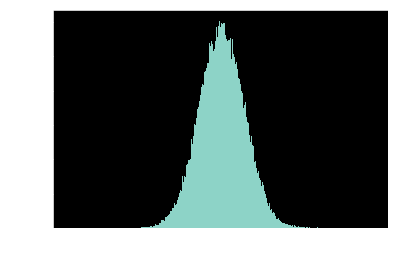### Step 2: Compute the number of photoelectrons¶

At this point we're going to need some numbers for our camera. For this example, I chose a camera using the popular Sony IMX264 CMOS chip, the FLIR Chamleon 3 (part number CM3-U3-50S5M-CS). Here are some of the key specs for our modeling:

Spec Value
Quantum efficiency (at 525 nm) 0.69
Temporal dark noise 2.29 e-
Pixel size 3.45 microns

Assuming our light has a wavelength of 525 nm, then the number of photoelectrons is simply the product of the quantum efficiency with the realization of the field.

In :
quantum_efficiency = 0.69
# Round the result to ensure that we have a discrete number of electrons
electrons = np.round(quantum_efficiency * shot_noise)

fig, (ax0, ax1) = plt.subplots(ncols=2)
img0 = ax0.imshow(shot_noise, vmin=200, vmax=600)
ax0.set_xticks([])
ax0.set_yticks([])
ax0.set_title('Photons')

divider = make_axes_locatable(ax0)
cb0 = plt.colorbar(img0, cax=cax)
cb0.set_ticks([])

img1 = ax1.imshow(electrons, vmin=200, vmax=600)
ax1.set_xticks([])
ax1.set_yticks([])
ax1.set_title('Electrons')

divider = make_axes_locatable(ax1)
cb = plt.colorbar(img1, cax=cax)

plt.show()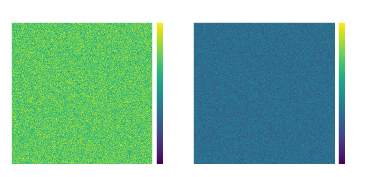### Step 3: Simulate read noise and dark current¶

Following the schematic of the camera model above, we see that we next need to add the dark noise. Dark noise (not to be confused with dark current) refers to the noise in the pixels when there is no light on the camera. Typically we deal with two sources of dark noise: readout (or just "read") noise and dark current. The camera specs in our example only provide us with the total dark noise, which is not surprising since dark current is not very significant in microscopy or machine vision. (It is however significant in astronomy because of the very long exposure times of several seconds or more, which allows appreciable dark current electrons to accumulate during the exposure.)

To the best of my knowledge, when dark noise is primarily read noise we may well model it as a Gaussian distribution whose standard deviation is equivalent to the dark noise spec of the camera. This modeling approach is taken, for example, in the 2016 SMLMS Challenge, a community-driven competition to identify the best reconstruction algorithms for single molecule localization microscopy. Please note that the noise model employed in the challenge was for an electron multiplying CCD (EMCCD), so you may find that it differs slightly from what I am discussing here.

In :
dark_noise = 2.29 # electrons
electrons_out = np.round(rs.normal(scale=dark_noise, size=electrons.shape) + electrons)

fig, (ax0, ax1) = plt.subplots(ncols=2)
img0 = ax0.imshow(electrons, vmin=250, vmax=450)
ax0.set_xticks([])
ax0.set_yticks([])
ax0.set_title('Electrons In')

divider = make_axes_locatable(ax0)
cb0 = plt.colorbar(img0, cax=cax)
cb0.set_ticks([])

img1 = ax1.imshow(electrons_out, vmin=250, vmax=450)
ax1.set_xticks([])
ax1.set_yticks([])
ax1.set_title('Electrons Out')

divider = make_axes_locatable(ax1)
cb = plt.colorbar(img1, cax=cax)
cb.set_label('Electrons')

plt.show()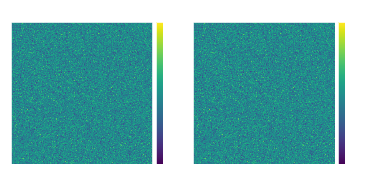In :
# Plot the difference between the two
fig, ax = plt.subplots()
img = ax.imshow(electrons - electrons_out, vmin=-10, vmax=10)
ax.set_xticks([])
ax.set_yticks([])
ax.set_title('Difference')

cb = plt.colorbar(img)
cb.set_label('Electrons')

plt.show()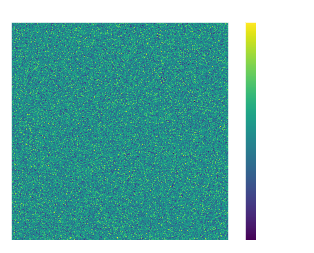### Step 4: Convert from $e^-$ to ADU¶

Now we need to convert the value of each pixel from electrons to ADU, or analog-to-digital units. ADU's are the units of grey scale that are output by monochrome cameras. To do this, we multiply the number of electrons after the addition of read noise by the sensitivity. We also need to ensure that the final ADU count is discrete and to set the maximum upper value of ADU's to the $2^k - 1$ where $k$ is the camera's bit-depth. The latter operation ensures that saturation of the pixels is correctly modeled.

In :
sensitivity = 5.88 # ADU/e-
bitdepth    = 12

fig, ax = plt.subplots()
ax.set_xticks([])
ax.set_yticks([])

cb = plt.colorbar(img)

plt.show()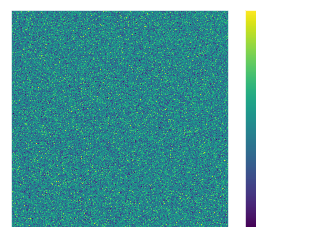### Step 5: Add a basline¶

Many cameras have a built-in baseline ADU value. This prevents the number of ADU's from becoming negative at low input signal. The Andor Zyla 4.2, for example, has a baseline value of 100 ADU. If you take a large number of dark frames and compute the average of each pixel in a Zyla, the average values will very nearly be 100.

We don't have baseline information for this camera, but 100 seems like a reasonable assumption. Here's what our final image will look like if we had a constant average of 500 photons incident on each pixel.

In :
baseline  = 100 # ADU

# EDIT: Note that we could have easily just performed this next step once
# here, rather than in the previous step

fig, ax = plt.subplots()
ax.set_xticks([])
ax.set_yticks([])

cb = plt.colorbar(img)

plt.show()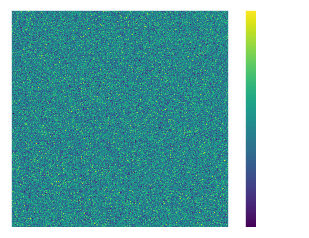## Adding noise to a simulated hologram¶

Let's see how the hologram from my last post will look like if I add camera noise to it. I'm going to assume that we are using the same camera as in my example to record the hologram. First, we'll setup the Fourier optics code as before to make the hologram. Then, we'll write a function that adds the noise and, in each frame of the animation, call it with the calculated field as an input.

This first part more-or-less repeats the essential bits of my last post. We make a circular object that doesn't absorb any light but which has a refractive index which causes a constant phase shift of 0.75 radians. We then use Fourier optics to compute the scattered field in the same plane as the object; this will be the starting frame of the animation. Instead of directly computing the number of photons per pixel from the field, we simply renormalize it so that the maximum value is 100 photons. 100 photons might seem like a weak signal, but this is actually quite a good camera and we will need to make the signal weak so that we can see the noise in the movie. In fact, the spec sheet for the camera reports an absolute minimum detectable signal of about 4 photons per pixel.

In :
# Ground truth: a circular phase-only object
numPixels = 512
gtCenter  = numPixels / 2 # assumes numPixels is even
W, H      = np.meshgrid(np.arange(0, numPixels), np.arange(0, numPixels)) # coordinates of the array indexes
gtMask    = np.sqrt((W - gtCenter)**2 + (H - gtCenter)**2) <= gtRadius # boundaries of the object
gt = np.ones((numPixels, numPixels), dtype=np.complex)

# Physical dimensions and sampling
pixelSize = 0.1 # microns
x = np.linspace(-pixelSize * numPixels / 2, pixelSize * numPixels / 2, num = numPixels, endpoint = True)

dx = x - x    # Sampling period, microns
fS = 1 / dx         # Spatial sampling frequency, inverse microns
df = fS / numPixels # Spacing between discrete frequency coordinates, inverse microns
fx = np.arange(-fS / 2, fS / 2, step = df) # Spatial frequency, inverse microns

# Fourier transform of ground truth
GT = ifftshift(fft2(fftshift(gt))) * dx**2

# Angular spectrum propagator and spatial frequencies
def H(fx, fy, z, wavelength=0.5):
square_root = np.sqrt(1 - (wavelength**2 * fx**2) - (wavelength**2 * fy**2))
temp = exp(1j * 2 * np.pi * z / wavelength * square_root)
temp[np.isnan(temp)] = 0 # replace nan's with zeros
return temp

FX, FY = np.meshgrid(fx, fx)

# Field at a distance of z=0
gt_prime = fftshift(ifft2(ifftshift(GT))) / dx**2

# Normalizing constant: makes the maximum photon count at z = 0 equal to 100 photons
norm = np.max(np.abs(gt_prime)**2) / 100


Next we create a Python function which takes as input a map of photons per pixel from our Fourier optics calculations and our camera specs. Since it doesn't have a large effect, I am skipping the explicit rounding of continuous numbers at each step and saving it until the end when the ADU's are calculated. I also added a random number generator to the inputs in case you want to reproduce previous results, but this isn't too important for this post.

In :
# Function to add camera noise
dark_noise=2.29, bitdepth=12, baseline=100,
rs=np.random.RandomState(seed=42)):

# Convert to electrons
electrons = qe * photons

electrons_out = rs.normal(scale=dark_noise, size=electrons.shape) + electrons

adu         = (electrons_out * sensitivity).astype(np.int) # Convert to discrete numbers



Finally, we create our animation of the simulated digital images of an inline planewave hologram from the object at axial planes between $0$ and $100 \, \mu m$ away from the object. It's true that some of the noise you will see in the video is due to the compression artifacts, but a lot of it is also due to the temporal noise in each individual pixel. Pay close to attention the regions of low signal to see it.

In :
# Create the animation as before, this time add camera noise
numPoints = 100
z         = np.linspace(0, 100, num = numPoints)

fig, ax    = plt.subplots(nrows=1, ncols=1)
vmin, vmax = 0, 1500

img = ax.imshow(np.abs(gt_prime)**2 / norm, vmin=vmin, vmax=vmax)
img.set_extent([x, x[-1], x, x[-1]])
ax.set_xticks([x, x[-1]])
ax.set_yticks([x, x[-1]])
ax.set_xlabel(r'$x, \, \mu m$')

cb  = plt.colorbar(img)
cb.set_clim([vmin, vmax])

txt = ax.text(8, 20, 'z = {:.1f} $\mu m$'.format(0))

# Initialize the figure with an empty frame
def init():
img.set_data(np.zeros((numPixels, numPixels)))
return img,

# This function is called for each frame of the animation
def animate(frame):
gt_prime = fftshift(ifft2(ifftshift(GT * H(FX, FY, z[frame], wavelength=0.525)))) / dx**2

# Divide by norm to convert to a photons
hologram = np.abs(gt_prime)**2 / norm

img.set_extent([x, x[-1], x, x[-1]])

txt.set_text('z = {:.1f} $\mu m$'.format(z[frame]))
return img,

# Create the animation
anim = animation.FuncAnimation(fig, animate, frames=numPoints,
init_func=init, interval=20, blit=True)

# Save the animation
myWriter = animation.FFMpegWriter(fps=10, extra_args=['-vcodec', 'libx264'])
anim.save('propagating_hologram_with_noise.mp4', writer = myWriter)

plt.close()

/home/kmdouglass/venvs/nikola/lib/python3.7/site-packages/ipykernel_launcher.py:25: RuntimeWarning: invalid value encountered in sqrt


## Simulated holograms on a poor camera¶

As I mentioned, the FLIR Chameleon camera is actually quite good. In fact, a similar camera with the Sony IMX265 chip has been successfully used for single molecule localization microscopy, which means that the noise floor is low enough and the quantum efficiency is high enough to detect the fluorescence from single molecules. Here's what another hologram might look like if the dark noise was worse, say 20 electrons. (For comparison, many sensors in digital cameras have read noise values around or below 10 electrons. Thanks @kD3AN for this link.)

Notice how many of the features of the hologram--especially those away from the center--become much more difficult to detect due to increased dark noise of the sensor. In particular, try pausing both movies around a distance of $40 \, \mu m$ and notice how much clearer the rings are for the low noise camera.

In :
# Create the animation as before, this time add camera noise
numPoints = 100
z         = np.linspace(0, 100, num = numPoints)

fig, ax    = plt.subplots(nrows=1, ncols=1)
vmin, vmax = 0, 1500

img = ax.imshow(np.abs(gt_prime)**2 / norm, vmin=vmin, vmax=vmax)
img.set_extent([x, x[-1], x, x[-1]])
ax.set_xticks([x, x[-1]])
ax.set_yticks([x, x[-1]])
ax.set_xlabel(r'$x, \, \mu m$')

cb  = plt.colorbar(img)
cb.set_clim([vmin, vmax])

txt = ax.text(8, 20, 'z = {:.1f} $\mu m$'.format(0))

# Initialize the figure with an empty frame
def init():
img.set_data(np.zeros((numPixels, numPixels)))
return img,

# This function is called for each frame of the animation
def animate(frame):
gt_prime = fftshift(ifft2(ifftshift(GT * H(FX, FY, z[frame], wavelength=0.525)))) / dx**2

# Divide by norm to convert to a preset number of photons
hologram = np.abs(gt_prime)**2 / norm

img.set_extent([x, x[-1], x, x[-1]])

txt.set_text('z = {:.1f} $\mu m$'.format(z[frame]))
return img,

# Create the animation
anim = animation.FuncAnimation(fig, animate, frames=numPoints,
init_func=init, interval=20, blit=True)

# Save the animation
myWriter = animation.FFMpegWriter(fps=10, extra_args=['-vcodec', 'libx264'])
anim.save('propagating_hologram_with_noise_poor.mp4', writer = myWriter)

plt.close()

/home/kmdouglass/venvs/nikola/lib/python3.7/site-packages/ipykernel_launcher.py:25: RuntimeWarning: invalid value encountered in sqrt


## Discussion¶

The addition of realistic camera noise to the hologram simulations is an important part of the simulation framework. It's impossible to record a digital hologram without noise, so any good holographic reconstruction algorithm needs to be capable of making estimates of the original object in the presence of shot noise and noise from the camera.

One source of noise that we did not model was noise associated with pixel non-uniformities. This sort of effect represents a pixel-dependent sensitivity, quantum efficiency, and dark noise. Non-uniformities are very often important take into account in image analysis but difficult to both measure and model. They're difficult to measure because you need a very stable light source that is very flat to a degree that is better than 99% across the camera chip. Believe me when I say that creating such a flat illumination pattern is extremely difficult, even with incoherent light. (The way to do it is to use an integrating sphere that directly faces the camera chip. The f-number of exit port of the sphere is recommended to be F/# = 8 in the EMVA 1288 standard.) They're difficult to model because they cannot be represented by a single number the way, for example, dark noise or quantum efficiency can.

The type of noise we modeled is called temporal noise because it deals with pixel-to-pixel variations in the output of the grey values. This is an important but often over-looked distinction when discussing camera noise.

Finally, the code I developed above should serve as a great tool to help you test cameras before you buy them. Most manufacturers will provide all the numbers used in the model on their websites, especially if they follow the EMVA 1288 Standard. Look up the numbers, plug them into the add_camera_noise function, and simulate a few frames to get a rough idea of how your images are going to look.

# Simulating Inline Holograms

Software has played a big role in digital holographic imaging, arguably starting from the publication in which Goodman and Lawrence generated images from electronically recorded holograms. One of the things I would like to do for my lensless imager project is to develop a small set of tools to simulate various types of digital holograms. My reason for wanting to do this is that it will help in developing the algorithms that will actually perform the reconstructions from real holograms. In this post, I will explore a simple method for simulating holograms that is explained in an excellent publication I recently found: Tatiana Latychevskaia and Hans-Werner Fink, " Practical algorithms for simulation and reconstruction of digital in-line holograms," arXiv 1412.3674 (2016).

## Inline holography with plane waves¶

### Create the ground truth¶

The first step in the simulation of a digital hologram is to create a ground truth object, i.e. a an object whose optical properties we know a priori. Rather than try to infer an object from a hologram--which is what the lensless imager should do--the simulations should work in the opposite sense and create a realistic hologram from the ground truth. Later, we can use the simulated holograms to test different reconstruction algorithms and see which ones come closest to reproducing the known objects.

In :
%pylab inline
from numpy.fft import fft2, fftshift, ifftshift
import scipy
from scipy.integrate import simps
from mpl_toolkits.axes_grid1 import make_axes_locatable
plt.style.use('dark_background')
plt.rcParams['figure.facecolor'] = '#272b30'
plt.rcParams['image.cmap'] = 'viridis'

print('Python version:\n{}\n'.format(sys.version))
print('Numpy version:\t\t{}'.format(np.__version__))
print('matplotlib version:\t{}'.format(matplotlib.__version__))
print('Scipy version:\t\t{}'.format(scipy.__version__))

Populating the interactive namespace from numpy and matplotlib
Python version:
3.5.2 |Continuum Analytics, Inc.| (default, Jul  2 2016, 17:53:06)
[GCC 4.4.7 20120313 (Red Hat 4.4.7-1)]

Numpy version:		1.11.3
matplotlib version:	2.0.0
Scipy version:		0.19.0


For the first simulation, I will start by making a relatively simple object: a non-absorbing circle with a constant phase. In holography, objects are represented by a position-dependent amplitude and phase. The amplitude determines how much the object absorbs the incident light and the phase determines by how much the light inside the object is slowed-down relative to the light that passes outside the object.

To create a non-absorbing circle, I create a square array of pixels whose values are all equal to one. What's neat about this is that this array already represents what is perhaps the simplest object to simulate: nothing at all! If every pixel is equal to one, it means that the light is neither being absorbed nor slowed down anywhere.

Of course, we want to eventually look at microscopic objects like cells, so we create a simple cell by making a circular mask and adjust the phase of all the pixels inside the mask relative to those outside it.

In :
numPixels = 512
gtCenter  = numPixels / 2 # assumes numPixels is even
W, H      = np.meshgrid(np.arange(0, numPixels), np.arange(0, numPixels)) # coordinates of the array indexes
gtMask    = np.sqrt((W - gtCenter)**2 + (H - gtCenter)**2) <= gtRadius # boundaries of the object

gt = np.ones((numPixels, numPixels), dtype=np.complex)


The last line of the code above, gt[gtMask] = np.exp(1j * gtPhase), sets all the values in the gt array that are inside the circular mask to the complex phasor $\exp \left( j \phi \right)$, where $\phi$ is the value of the phase imparted onto the light by the object. For real objects, this phase would be equal to the optical path length of the object, which is the product of the object's refractive index by its real size.

Let's now go ahead and visualize the object to make sure we created it correctly.

In :
def plot_complex_field(field, vmin=0, vmax=1):
fig, ax = plt.subplots(nrows=1, ncols=2)

amp   = ax.imshow(np.abs(field))
phase = ax.imshow(np.angle(field), vmin=0, vmax = 2*np.pi)

ax.set_xticks([])
ax.set_yticks([])
ax.set_title('Amplitude')

divider = make_axes_locatable(ax)
cb_amp = plt.colorbar(amp, cax=cax)

ax.set_xticks([])
ax.set_yticks([])
ax.set_title('Phase')

divider = make_axes_locatable(ax)
cb_phase  = plt.colorbar(phase, cax=cax)
cb_phase.set_ticks([0, 2*np.pi])
cb_phase.set_ticklabels(['0', r'2$\pi$'])

return fig, ax, amp, phase, cb_amp, cb_phase

In :
_, _, _, _, cb_amp, _ = plot_complex_field(gt)
cb_amp.set_ticks()
plt.show()As you can see, the amplitude remains equal to 1 everywhere, which is what we would expect for a non-absorbing object. In the plot of the phase you can see the faint circle at the center of the image. I plotted the phase on a scale of $\left[ 0, 2 \pi \right]$ for two reasons:

1. to emphasize that the object only weakly perturbs the light;
2. because one complete rotation of the phasor in the complex plane is $2 \pi$ radians. Any value outside the range $\left[ 0, 2 \pi \right]$ can be mapped back onto a value in this range.

#### Set the physical dimensions¶

The final step in the object creation is to set up the physical dimensions of the problem. There are a few ways we can do this, but I typically like to set the number of pixels that sample the object and the pixel size independently. Then, the physical radius of the object is equivalent to gtRadius * pixelSize and the total array size is equal to numPixels * pixelSize. What's more, because the array is square, we can create just one coordinate vector for both directions.

In :
pixelSize = 0.1 # microns
x = np.linspace(-pixelSize * numPixels / 2, pixelSize * numPixels / 2, num = numPixels, endpoint = True)


The physical radius is:  5.0 microns


The computational tool that I will use to propagate the wave fronts is known as the angular spectrum propagator. This tool requires two Fourier transforms and therefore requires that I setup the arrays that denote the spatial frequencies as well. As I discussed in a previous post, this can be done like so:

In :
dx = x - x    # Sampling period, microns
fS = 1 / dx         # Spatial sampling frequency, inverse microns
df = fS / numPixels # Spacing between discrete frequency coordinates, inverse microns
fx = np.arange(-fS / 2, fS / 2, step = df) # Spatial frequency, inverse microns


dx is the sampling period in the object space, whose inverse fS is the sampling frequency. The maximum information content in the object's Fourier transform is found at the Nyquist frequency, or fS / 2.

### Compute the hologram¶

Two common ways to generate a hologram in a real experiment are to illuminate the object with a plane wave and with a spherical wave. In this simulation, I will assume a plane wave illumination because this is somewhat simpler and an easy first case to deal with.

#### Step 1: Find the Fourier transform of the object¶

According to the publication by Latychevskaia and Fink, we first need to compute the Fourier transform of the object.

In :
GT = ifftshift(fft2(fftshift(gt))) * dx**2
fig, ax, amp, phase, cb_amp, _ = plot_complex_field(GT)

amp.set_extent([fx, fx[-1], fx, fx[-1]])
ax.set_xticks([-fS / 2, fS / 2])
ax.set_yticks([-fS / 2, fS / 2])
ax.set_xlabel(r'$f_{x}, \, \mu m^{-1}$')
ax.set_ylabel(r'$f_{y}, \, \mu m^{-1}$')
cb_amp.set_ticks([0, 2612]) # I think that there's a bug in matplotlib 2.0 preventing correct tick displays

phase.set_extent([fx, fx[-1], fx, fx[-1]])
ax.set_xticks([-fS / 2, fS / 2])
ax.set_yticks([-fS / 2, fS / 2])
ax.set_xlabel(r'$f_{x}, \, \mu m^{-1}$')

plt.tight_layout()
plt.show()We cannot really see much in the amplitude plot, so let's put it on a log-scale.

In :
fig, ax = plt.subplots(nrows=1, ncols=1)

img = ax.imshow(np.log10(np.abs(GT)), extent=[fx, fx[-1], fx, fx[-1]])
cb  = plt.colorbar(img)
cb.set_label('$\log_{10}$ amplitude')

ax.set_xticks([-fS / 2, fS / 2])
ax.set_yticks([-fS / 2, fS / 2])
ax.set_xlabel(r'$f_{x}, \, \mu m^{-1}$')
ax.set_ylabel(r'$f_{y}, \, \mu m^{-1}$')

plt.show()